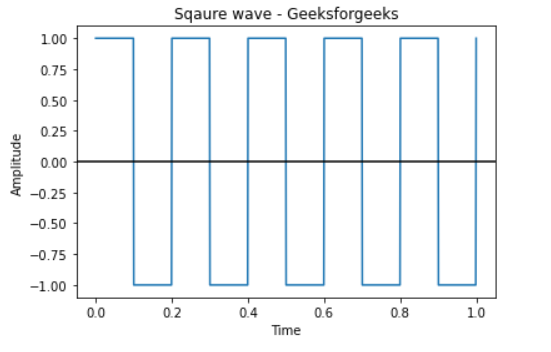# Plotting A Square Wave Using Matplotlib, Numpy And Scipy

• Last Updated : 20 Apr, 2022

Prerequisites: linspace, Mathplotlib, Scipy

A square wave is a non-sinusoidal periodic waveform in which the amplitude alternates at a steady frequency between the fixed minimum and maximum values, with the same duration at minimum and maximum. Graphical representations are always easy to understand and are adopted and preferable before any written or verbal communication. In this article, we will try to understand, How can we plot Square waves using the Scipy python module.

Approach:

• Import required module.
• Create a sample rate.
• Plot a square wave.
• Label the graph.
• Display Graph.

Step 1: Import module

## Python3

 `from` `scipy ``import` `signal``import` `matplotlib.pyplot as plot``import` `numpy as np`

Step 2: The NumPy linspace function is a tool in Python for creating numeric sequences that return evenly spaced numbers over a specified interval.

## Python3

 `t ``=` `np.linspace(``0``, ``1``, ``1000``, endpoint ``=` `True``)`

Step 3: plot.plot function: This method accepts the following parameters and Plot the square wave signal.

Syntax:

scipy.signal.square(t)

Parameter:

• t: The input time array.

Return:

Output array containing the square waveform.

## Python3

 `# Plot the square wave``plot.plot(t, signal.square(``2` `*` `np.pi ``*` `5` `*` `t))`

Step 4: Give title name, x-axis label name, y-axis label name.

## Python3

 `# Give x,y, title axis label``plot.xlabel(``'Time'``)``plot.ylabel(``'Amplitude'``)``plot.title(``'Square wave - Geeksforgeeks'``)`

Step 5: plot.axhline() : The axhline() function in pyplot module of matplotlib library is used to add a horizontal line across the axis.

## Python3

 `# Provide x axis and black line color``plot.axhline(y``=``0``, color``=``'k'``)`

Below is the full implementation:

## Python3

 `from` `scipy ``import` `signal``import` `matplotlib.pyplot as plot``import` `numpy as np` `t ``=` `np.linspace(``0``, ``1``, ``1000``, endpoint``=``True``)``    ` `# Plot the square wave``plot.plot(t, signal.square(``2` `*` `np.pi ``*` `5` `*` `t))` `# Give x,y,title axis label``plot.xlabel(``'Time'``)``plot.ylabel(``'Amplitude'``)``plot.title(``'Square wave - Geeksforgeeks'``)` `plot.axhline(y ``=` `0``, color ``=` `'k'``)` `# Display``plot.show()`

Output:My Personal Notes arrow_drop_up